# \$B6d2O\$NNr;K\$,\$^\$?#1%Z!<%8(B(\$BF|5-(B)

Last Update (2001/10/23 00:58:16)
1997.09.06\$B\$+\$i?t\$(\$F(B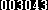\$BHVL\\$N%"%/%;%9\$G\$9!#(B

\$B%_%i!<%5%\$%H(B [www.ceres.dti.ne.jp] [yk.rim.or.jp]

### \$B"#(B \$B1'ChNr(B 2001.10.01 (Mon)

http://www.ceres.dti.ne.jp/~george/jdiaryA11001.html#20011001

#### \$B\$\$\$m\$\$\$m(B

apache\$B\$N%m%0\$N<+F02sE>(B\$B\$rJd40\$7\$?\$j!#(B AC4\$B\$G(BS RANK\$B%W%l%\$\$7\$F\$?\$j!#8=:_%_%C%7%g%s(B8\$B\$^\$G(BS RANK\$B\$G\$-\$?!#(B

### \$B"#(B \$B1'ChNr(B 2001.10.02 (Tue)

http://www.ceres.dti.ne.jp/~george/jdiaryA11001.html#20011002

#### HTML::del, ins \$BMWAG(B

ArctanX\$B\$5\$s\$H\$3(B\$B\$+\$i\$?\$I\$C\$F(B\$B\$4\$/4JC1\$J(BHTML\$B\$N@bL@(B\$B!#(B

ins, del \$B\$J\$s\$FMWAG\$"\$C\$?\$N\$+!D!#(B \$B\$I\$l\$I\$l!#(B \$BAaB.I=<(\$7\$F\$_\$k%F%9%H!#(B

\$B:o=|(B\$BDI2C(B

Mozilla-0.9.4/IE 5.5 SP2/IE 5.0 SP2\$B\$GI=<(\$5\$;\$F\$_\$?!#(B \$B\$3!<\$f! \$B\$A\$J\$_\$K!"%&%A\$N(Bwwwoffle\$B\$N%-%c%C%7%eFbIt\$r8!:w\$7\$?\$H\$3\$m!"(B<ins> / <del> \$B\$r;H\$C\$F\$\$\$kJ}\$O(B1\$BL>\$7\$+\$\$\$J\$+\$C\$?\$j!#(B

\$B\$D\$\$\$G\$K!"(Binline\$B\$J0zMQ\$r\$"\$i\$o\$9(B q \$BMWAG\$b%A%'%C%/!#(B \$B\$3\$l\$O@oAh\$@(B\$B\$H\$@\$l\$=\$l\$O8@\$C\$?!#(B \$B\$U\$`\$U\$`!#(BMozilla\$B\$@\$H(B " \$B\$G0O\$^\$l\$k\$,(B IE \$B\$G\$OFC\$KJQ2=\$J\$7!"\$H!#(B

#### Solaris 8 Source \$B\$-\$?\$h(B

\$B@hF|(B\$BCmJ8\$7\$F\$\$\$?(BSolaris[tm] 8 Foundation Source Program \$B\$,E~Ce!#(B \$B\$^\$@L\$3+Iu\$@\$1\$I!#(B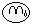### \$B"#(B \$B1'ChNr(B 2001.10.04 (Thu)

http://www.ceres.dti.ne.jp/~george/jdiaryA11001.html#20011004

#### RE:DynDNS\$B\$G(BVirtualHost

\$B;d\$b\$[\$H\$s\$I%N!<%A%'%C%/\$J\$N\$G\$9\$,!"(BDynDNS\$B\$G(BVirtualHost\$B\$OB?J,\$G\$-\$k\$s\$8\$c\$J\$\$\$+\$J!A\$H;W\$\$\$^\$9!#(B DynDNS\$B\$O\$J\$s\$H\$J\$/(BCNAME\$B\$N@_Dj(B(Wildcard\$B\$r(BON\$B\$K\$9\$k\$H!"(B*.ore-server.homeip.net\$B\$,A4ItF1\$8(BIP\$B%"%I%l%9\$r;X\$9@_Dj(B)\$B\$,2DG=\$G\$9\$7!#(B \$B\$"\$H\$O!"(BNameVirtualHost * \$B\$r5-F~\$7\$F!"(BVirtualHost\$B\$r=q\$1\$P\$J\$s\$H\$+\$J\$k\$s\$8\$c\$J\$\$\$+\$H(B....\$B!#(B

#### FreeBSD::Wind River\$B\$,(BFreeBSD\$B4X785;=Q

Daily Daemon News\$B\$+\$i(BWindRiver lays off FreeBSD staffers\$B\$X!#(B IT\$B6H3&\$N(B(IT\$B6H3&\$K8B\$i\$s\$1\$I(B)\$BIT67\$O?<9o\$G\$9\$o!#(B FreeBSD Project\$B\$N9T\$/Kv\$,ITF)L@\$K\$J\$C\$F\$\$\$k\$N\$+\$7\$i\$s!#(B \$B>e5-\$N%9%l%C%I\$+\$i(Bjkh\$B\$5\$s\$NH/8@\$r0ULu!#(B \$B\$^\$A\$,\$C\$F\$?\$i%D%C%3%_\$h\$m\$7\$&(B(\$B\$)!#(B

``` First, I don't think that anyone should be surprised to see
WindRiver, or any other company for that matter, making certain
difficult financial decisions in the current economic climate. Unless
you've been living under a rock these last 12 months, you know that
business sucks and a lot of really good people have been getting laid
off left and right in the IT industry. To expect an open source
development group like this to be spared the same treatment just
wouldn't be very realistic right now.

\$B8=:_\$N7P:Q>u67\$K\$*\$\$\$F\$O!"(BWindRiver\$B\$d\$=\$NB>\$N\$I\$s\$J4k6H\$G\$b!":#2s\$N\$h\$&(B
\$B\$J7P1D>e\$NFq\$7\$\$7hCG\$r\$9\$k\$3\$H\$KBP\$7\$F6C\$/\$Y\$-\$G\$O\$J\$\$!#\$3\$3:G6a(B12\$B%u7n\$N(B
\$B4V\$:\$C\$H4d\$N2<\$GJk\$i\$7\$F\$\$\$k?M\$G\$9\$i!"(BIT\$B;:6H\$K\$*\$\$\$F\$O%S%8%M%9\$O9T\$-5M\$^(B
\$B\$j!"Bt;3\$NK\Ev\$KNI\$\$?MC#\$,%l%\$%*%U\$5\$l\$D\$D\$"\$k\$3\$H\$rCN\$C\$F\$\$\$k!#%*!<%W%s(B
\$B%=!<%93+H/%0%k!<%W\$@\$1\$,FCJL07\$\$\$5\$l\$k\$3\$H\$O\$"\$j\$(\$J\$\$!#(B
```
```What's more important is for people to understand how or if this will
a painless event by any means, least of all for the people involved,
it's also something that the FreeBSD project can and will deal with.

\$B?M!9\$K\$H\$C\$F\$h\$j=EMW\$J\$3\$H\$O!"\$3\$N;v7o\$,(BFreeBSD project\$B\$K1F6A\$rM?\$(\$k\$+(B
\$B\$I\$&\$+!"M?\$(\$k\$H\$9\$l\$P\$I\$s\$J1F6A\$rM?\$(\$k\$+\$rM}2r\$9\$k\$3\$H\$@!#\$3\$N;v7o\$ODK(B
\$B\$_\$,L5\$\$\$o\$1\$G\$O\$J\$\$\$,!"(BFreeBSD project\$B\$,07\$&\$3\$H\$,\$G\$-\$k!"07\$o\$J\$1\$l\$P(B
\$B\$J\$i\$J\$\$LdBj\$@!#(B
```
```The FreeBSD project has had a long history of making a lot happen with
very little resources, both man and machine, and a track record I
think we can all be proud of where it comes to dealing with the many
temporary set-backs that have occurred during almost 10 years of
continuous evolution. Even subtracting WindRiver's contribution, the
project still has a lot of developers working almost continuously on
FreeBSD and the sustained backing of numerous companies. Yahoo!
continues to site many of the project's machines and donate resources,
as does Qwest, and many of the other companies and individuals one
finds listed in the FreeBSD gallery are still putting their time and
energy behind the project. Taken in the context of these recessionary
times, I'm actually somewhat amazed at how well the project is
currently doing.

FreeBSD project\$B\$OHs>o\$K>/\$J\$\$?ME*!"5!3#E*%j%=!<%9\$GBt;3\$N;E;v\$r@.\$7?k\$2(B
\$B\$FMh\$?D9\$\$Nr;K\$,\$"\$k!#\$=\$7\$F2f!9\$O(B10\$BG/\$K\$o\$?\$k?J2=\$NCf\$G!"B?\$/\$N0l;~E*\$J(B
\$B8eB`\$KBP=h\$7\$FMh\$?\$3\$H\$r8X\$j\$K;W\$C\$FNI\$\$!#\$?\$H\$((BWindRiver\$B\$N9W8%\$,L5\$/\$J\$C(B
\$B\$?\$H\$7\$F\$b!"(B(FreeBSD) project\$B\$O3+H/\$rB3\$1\$kB?\$/\$N3+H/l=j\$rDs6!\$7!"%j%=!<%9\$N(B
\$B4sIU\$rB3\$1\$F\$*\$j!"(BQwest\$B\$bF1MM\$@!#(BFreeBSD \$B%.%c%i%j!<\$KL>\$rO"\$M\$kB?\$/\$N4k(B
\$B6H\$d8D?M\$O(B(FreeBSD) project\$B\$K%(%M%k%.!<\$H;~4V\$rCm\$.B3\$1\$F\$\$\$k!#:G6a\$N7J(B
\$B5\$8eB`\$r9MN8\$9\$l\$P!"(Bproject\$B\$,8=:_Hs>o\$K\$&\$^\$/9T\$C\$F\$\$\$k\$3\$H\$K\$\$\$/\$i\$+\$N(B
\$B6C\$-\$r3P\$(\$k!#(B
```
```WindRiver's contributions have certainly been appreciated, and I'm
sure many of us hope that we can perhaps find various ways of
collaborating again in the future, but it still bears noting that the
FreeBSD project was around and doing quite well long before WindRiver
arrived and, \$deity willing, will be around long after today's events.

WindRiver\$B\$N9W8%\$O3N\$+\$KI>2A\$G\$-\$k\$7!";d\$O2f!9\$NCf\$NB?\$/\$N?M!9\$,>-Mh:F\$S(B
\$B0l=o\$K:n6H\$G\$-\$k\$h\$&\$JJ}K!\$rH/8+\$G\$-\$k\$HK>\$s\$G\$\$\$k\$H3N?.\$7\$F\$\$\$k!#\$7\$+\$7!"(B
FreeBSD project\$B\$O@N\$+\$i\$"\$C\$?\$7!"(BWindRiver\$B\$,Mh\$k\$:\$C\$HA0\$+\$i\$&\$^\$/\$d\$C\$F(B
\$B\$-\$?\$7(B--\$B?@\$N2C8n\$r(B--\$B:#F|\$N=PMh;v\$+\$i\$:\$C\$H8e\$^\$G\$b\$"\$k\$@\$m\$&\$3\$H\$r9M\$(\$k(B
\$B\$H!"FCJL\$JJQ2=\$OL5\$\$\$@\$m\$&!#(B(\$BLuCp(B:deity willing\$B\$O\$J\$s\$+\$N8@\$\$2s\$7\$+\$J!)(B
)
```
```It is also clearly understood that certain questions still remain
unanswered by this message and that the FreeBSD user base will want
example, and will it now be transferred to the FreeBSD Foundation?
What will become of the FreeBSD CDROM and book publishing business
that was indirectly purchased from Walnut Creek CDROM? What will
become of the various test machines (e.g. non-critical but still
important resources) that were sited at WindRiver's Alameda campus?

\$BL@Gr\$@\$,!"\$3\$N%a%C%;!<%8(B(\$BLuCp(B:jkh\$B\$5\$s\$NH/8@\$N>e\$K\$"\$k85H/8@(B)\$B\$G\$O!"(B
FreeBSD\$B%f!<%6!<\$bEz\$(\$rK>\$s\$G\$\$\$k\$"\$k5?Ld\$K\$D\$\$\$F\$N2sEz\$,\$^\$@\$J\$\$!#\$?\$H(B
\$B\$(\$P!"(BFreeBSD\$B\$N>&I8\$O\$I\$&\$J\$k\$N\$+!">&I8\$O(BFreeBSD Foundation\$B\$K0\\$k\$3\$H\$K(B
\$B\$J\$k\$N\$+!)(BWalnut Creek CDROM\$B\$+\$i4V@\E*\$K9XF~\$7\$?(BFreeBSD CDROM\$B\$d=q@R\$N=P(B
\$BHG%S%8%M%9\$O\$I\$&\$J\$k\$N\$+!)(BWindRiver\$B\$N(BAlameda\$B%-%c%s%Q%9\$K\$"\$k\$5\$^\$6\$^\$J%F(B
\$B%9%H%^%7%s(B(\$BCWL?E*\$G\$O\$J\$\$\$,!"\$d\$O\$j=EMW\$J%j%=!<%9(B)\$B\$O\$I\$&\$J\$k\$N\$+!)(B
```
```I hope that WindRiver will assist us by addressing some of these
issues publically and, if and where it does not, you may rest assured
that others in the project will be posing such questions to the
appropriate people and will share the answers with the wider community
once they have them. For now, please try to recognize the fact that
dealing with the employees RIF'd has taken precedence over grappling
with these sorts of issues (and understandably so) so a little
patience is in order. It will take some extra time to tie up all the
loose ends here. Thanks.

\$B;d\$O!"(BWindRiver\$B\$,\$3\$l\$i\$NLdBj\$K\$D\$\$\$F\$*\$*\$d\$1\$KOC\$7!"2f!9\$r=u\$1\$FM_\$7\$\$(B
\$B\$HK>\$s\$G\$\$\$k!#\$?\$H\$((BWindRiver\$B\$,\$=\$&\$7\$J\$/\$H\$b!"(Bproject\$B\$K\$\$\$k?M!9\$,\$7\$+\$k(B
\$B\$Y\$-?M\$K/!9\$NG&(B
\$BBQ\$,I,MW\$K\$J\$k\$,!"\$3\$N/!9;~4V\$,\$+\$+\$k\$@\$m\$&!#(B(\$BLuCp(B:A
has taken precedence over B\$B\$G!"(BA\$B\$h\$j\$b(BB\$B\$NJ}\$,M%@h!"\$G\$"\$C\$F\$k\$h\$M!)\$b\$7(B
\$B5U\$@\$HLu\$,@5H?BP\$N0UL#\$K(B...(\$B\$)(B)\$B!#(B)
```

Wind River Systems\$B\$OAH\$_9~\$_%7%9%F%`\$J\$I\$r:n@.\$7\$FHNGd\$7\$F\$\$\$k2q.\$G6HL3\$O7QB3\$9\$k\$N\$+!"\$=\$l\$H\$b:#8e(BFreeBSD\$B\$H&I8\$HHNGd8"\$J\$s\$@\$7!"2q

#### GAME::AC4::\$BA4%_%C%7%g%s(BS RANK\$B\$X\$NF;(B

Mission 9\$B\$,(BS RANK\$Be967b\$C\$FFq\$7\$\$\$h\$&!#(B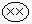### \$B"#(B \$B1'ChNr(B 2001.10.05 (Fri)

http://www.ceres.dti.ne.jp/~george/jdiaryA11001.html#20011005

#### Wind River Systems\$B\$N%l%\$%*%U\$NOC\$N(Bjkh\$B\$5\$s\$N%3%a%s%H\$r0ULu\$7\$F\$_\$?\$j(B

\$B:rF|%M%?(B\$B\$r0ULu\$7\$F\$_\$?\$j!#(B

#### WikiWiki clone - UseModWiki

perl\$B\$G=q\$+\$l\$?(BWiki Wiki clone\$B\$N(BUseModWiki v 0.92\$B\$r%@%&%s%m!<%I\$7\$F\$_\$?!#(B Wiki Wiki(\$B\$3\$3\$,K\2H\$+\$J!)(B)\$B\$H\$O!"C/\$G\$bJT=8\$G\$-\$k(BWeb\$B%Z!<%8\$rl9g\$J\$s\$+\$KJXMx\$i\$7\$\$!#(B \$BM#0l\$N\$B\$K\$0\$k\$5\$s\$NF|5-\$N\$I\$3\$+(B(\$B4@(B;)\$B\$G8+\$+\$1\$F!V%X%s\$JL>A0\$@\$J\$*\$\$!W\$H5-21\$K;D\$C\$F\$\$\$?\$N\$G%m!<%+%k%^%7%s\$K%\$%s%9%H!<%k\$7\$F\$_\$k!#(B

\$B<+F0E*\$KJT=8MzNr\$,;D\$C\$F!"(Bdiff\$B\$b
\$B%*%l\$OIaCJF|5-\$r=q\$/;~\$K(BHTML\$B\$N%?%0\$r \$B\$*\$C\$H!"F|K\8l\$rF~NO\$9\$k\$H2=\$1\$k\$M\$'!#(B jcode.pl\$B\$r\$V\$A\$3\$s\$G\$_\$k\$+\$J(B(\$B\$)!#(B
....\$B!#(B
.......\$B!#(B
UseModWiki\$B\$OFbIt\$G%j%s%/=hM}MQ\$N%U%#!<%k%I%;%Q%l!<%?\$H\$7\$F(B 0xb3 \$B\$r;H\$C\$F\$^\$9\$J\$!!#(B EUC-JP\$B\$K\$7\$?>l9g\$O(B0x90\$B\$r;H\$C\$FCV\$-49\$(\$F\$bJ?5\$\$+\$J!)(B \$B\$"\$H\$O(Bwiki.pl\$B\$N(Bcharset\$B\$r(BEUC-JP\$B\$K@_Dj\$7\$F!#(B
....\$B!#(B
wiki.pl\$B\$+\$i;H\$o\$l\$F\$\$\$k(BPerl\$B\$N%b%8%e!<%k(B CGI::\$B%7%j!<%:\$C\$F!"FI9~\$_;~\$K%3!<%IJQ49\$+\$1\$F\$J\$\$\$J!D!#(B \$B%U%)!<%`\$+\$iF~NO\$5\$l\$kJ8;z\$r(BEUC-JP\$B\$KE}0l\$7\$?\$\$>l9g\$O!D!#(B
....\$B!#(B
....\$B!#(B
\$B\$o\$+\$i\$s!#(B \$B\$H\$\$\$&\$o\$1\$G!"B.96\$GF|K\8l2=\$r:C@^\$7\$F\$_\$?\$j!#(B\$B0l1~!"(BHTTP\$B%X%C%@It\$K(Bcharset=EUC-JP\$B\$C\$F=q\$\$\$F\$"\$j!"(BWeb\$B%5!<%P\$K(Bapache\$B\$r;H\$C\$F\$\$\$k\$H!"(BMozilla-0.9.4\$B\$H\$+(BIE 5.0SP2\$B\$+\$i=q\$\$\$?>l9g\$O(BEUC-JP\$B\$G%F%-%9%H\$,Aw?.\$5\$l\$kLOMM!#(B \$B\$^\$H\$b\$KF0\$+\$J\$\$%V%i%&%6B??t\$NM=46\$@\$1\$I(B(\$B\$)!#(B

\$B0l1~:9J,!#(B

```--- wiki.pl	Sun Apr 22 09:44:10 2001
+++ wiki.cgi	Sat Oct  5 23:18:45 2001
@@ -55,12 +55,12 @@
\$q \$Now \$UserID \$TimeZoneOffset \$ScriptName \$BrowseCode \$OtherCode);

# == Configuration =====================================================
-\$DataDir     = "/tmp/mywikidb"; # Main wiki directory
+\$DataDir     = "/home/george/public_html/cgi-bin/usemodwiki/data/mywikidb"; # Main wiki directory
\$UseConfig   = 1;       # 1 = use config file,    0 = do not look for config

# Default configuration (used if UseConfig is 0)
\$CookieName  = "Wiki";          # Name for this wiki (for multi-wiki sites)
-\$SiteName    = "Wiki";          # Name of site (used for titles)
+\$SiteName    = "Wiki-test";     # Name of site (used for titles)
\$RCName      = "RecentChanges"; # Name of changes page (change space to _)
\$LogoUrl     = "/wiki.gif";     # URL for site logo ("" for no logo)
@@ -82,7 +82,7 @@
\$EditNote    = "";              # HTML notice above buttons on edit page
\$MaxPost     = 1024 * 210;      # Maximum 210K posts (about 200K for pages)
\$NewText     = "";              # New page text ("" for default message)
-\$HttpCharset = "";              # Charset for pages, like "iso-8859-2"
+\$HttpCharset = "EUC-JP";        # Charset for pages, like "iso-8859-2"
\$UserGotoBar = "";              # HTML added to end of goto bar

# Major options:
@@ -164,7 +164,7 @@
my (\$UpperLetter, \$LowerLetter, \$AnyLetter, \$LpA, \$LpB, \$QDelim);

# Field separators are used in the URL-style patterns below.
-  \$FS  = "\xb3";      # The FS character is a superscript "3"
+  \$FS  = "\x90";      # The FS character is a magic-number for EUC-JP
\$FS1 = \$FS . "1";   # The FS values are used to separate fields
\$FS2 = \$FS . "2";   # in stored hashtables and other data structures.
\$FS3 = \$FS . "3";   # The FS character is not allowed in user data.
```

wiki.pl\$BFbIt\$K\$O(B\$NonEnglish\$B\$H\$\$\$&@_Dj%U%i%0\$,\$"\$k\$1\$I!"L?CfH=Dj\$,(B

```  if (\$NonEnglish) {
\$UpperLetter .= "\xc0-\xde";
\$LowerLetter .= "\xdf-\xff";
\$AnyLetter   .= "\xc0-\xff";
}
```

\$B\$C\$F\$J46\$8\$J\$N\$G!"F|K\?M\$O;XDj\$7\$J\$\$J}\$,\$h\$5\$2!#(B

\$B\$A\$J\$_\$K!"K\2H(BWiki Wiki\$B\$O(BPython\$B\$G=q\$+\$l\$F\$*\$j!"2?\$b\$7\$J\$/\$F\$bF|K\8l\$J\$I\$KBP1~\$7\$F\$\$\$kLOMM!#(B

#### charset\$B\$G;H\$(\$k%Q%?!<%s(B

\$B\$4\$/4JC1\$J(BHTML\$B\$N@bL@(B\$B\$+\$iE,Ev\$KH4?h!#(B \$B@5<0\$J;2>H@h\$O(Bhttp://www.iana.org/assignments/character-sets\$B!#(B \$B>e\$N(BWiki Wiki\$B\$G\$A\$HG:\$s\$@\$N\$G&U(B(.. )\$B%a%b%a%b!#(B

```charset=iso-2022-jp
charset=Shift_JIS \$B\$^\$?\$O(B charset=x-sjis
charset=EUC-JP
```

\$B\$I\$&\$G\$b\$\$\$\$\$1\$I!"(BShift_JIS\$B\$O%"%s%@!<%P!<\$G(BEUC-JP\$B\$,%^%\$%J%9\$J\$N\$O2?8N\$J\$s\$@\$m\$&!)(B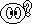#### Visor::Pocket Type

\$B\$D\$\$@hF|(BVisor\$BMQ\$N%-!<%\!<%I\$r9XF~\$7\$?%*%l\$K9s\$J%K%e!<%9!#(B \$B\$3\$s\$J?7@=IJ(BPocketType\$B\$,!#(B \$B\$&!<\$`!#(B \$B\$A\$g\$C\$HM_\$7\$\$5\$\$,\$9\$k\$s\$@\$1\$I!"A0\$N%-!<%\!<%I\$O@\B3%F%9%H\$H\$3\$s\$J\$sGc\$\$\$^\$7\$?%G%b%s%9%H%l!<%7%g%s\$N(B2\$B2s\$7\$+;H\$C\$F\$J\$\$\$+\$i\$J\$!!#(B#### BlueTooth\$B\$OGTKL\$7\$?!)(B

CNET \$B%V%k!<%H%%!<%9\$O4{\$KGTKL(B\$B\$J\$s\$F5-;v\$,!#(B \$B\$=!<\$\$\$d:G6aOCBj\$rJ9\$+\$J\$\$\$H;W\$C\$F\$?\$1\$I!"\$\$\$D\$N4V\$KGTKL\$7\$?\$s\$@\$m!)(B \$B%^%8!)(B

2001.10.09\$BDI5-!#(B Intel\$B\$NHNGd@oN,\$C\$]\$\$\$N\$GOCH>J,\$KJ9\$\$\$F\$*\$\$\$?J}\$,NI\$\$(B\$B\$H\$N%3%a%s%H\$,!#(B \$B\$I\$b\$G\$9!A!#(B

#### Solaris8 for Intel patch memo

SUN - Recommended Product Patches\$B\$K(BSolaris8 for Intel \$BMQ\$N%Q%C%AH/8+!#(B \$B\$\$\$\$\$+\$2\$s(BK6-200MHz\$B\$G(BSolaris8\$B;H\$&\$N\$O9iLd\$K6a\$\$\$7(B(\$B>P(B)\$B!"\$=\$m\$=\$m%^%7%s\$N%Q%o!<%"%C%W\$r9M\$(\$F\$O\$\$\$k\$s\$@\$1\$I!#(B \$B\$k5!3#\$C\$F(BAthlon-500\$B\$J\$s\$@\$h\$J!A!#(B Athlon\$B\$G(BSolaris8\$B\$OF0\$/\$N\$+!)(B\$B:G6aHNGd\$5\$l\$F\$\$\$k(BHDD\$B\$OL/\$KMFNL\$,Bg\$-\$\$\$N\$G!"%Q%C%A\$rF~\$l\$J\$\$\$H%^%:%\$\$=\$&\$H\$\$\$&\$3\$H\$G0l1~%A%'%C%/!#(B 40G over HDD\$BBP1~%Q%C%AH/8+!#(B 32G over HDD\$B\$K(BSolaris8\$B\$r?75,%\$%s%9%H!<%k\$9\$k;~\$G\$b!"%\$%s%9%H!<%i\$+\$i4hD%\$C\$FA`:n\$9\$l\$P?7%I%i%\$%P\$,;H\$(\$k\$i\$7\$\$!#(B \$B\$"\$k0UL#\$9\$2!

### \$B"#(B \$B1'ChNr(B 2001.10.07 (Sun)

http://www.ceres.dti.ne.jp/~george/jdiaryA11001.html#20011007

#### \$B\$*8+Iq(B

\$B\$^\$C\$?\$j\$H\$*8+Iq\$K9T\$C\$?\$j\$7\$F!#(B

#### NetBSD/dreamcast

\$B&U(B(.. )\$B%a%b%a%b!#(B

#### GAME::AC4::MISSION 9

Mission 9\$B\$O7k6I!"Bg7?L5M6F3GzCF\$rEk:\\$9\$k(BSu-37\$B\$G(BS RANK\$B%/%j%"!#(B \$B@:L)M6F3\$7\$J\$\$\$N\$GGzCF\$NO"\$_\$,\$"\$k!#(B \$B\$"\$H\$OHt\$s\$G\$\$\$kHt9T5!\$rA4It\$*\$H\$9\$D\$b\$j\$G4hD%\$l!A!#(B

### \$B"#(B \$B1'ChNr(B 2001.10.08 (Mon)

http://www.ceres.dti.ne.jp/~george/jdiaryA11001.html#20011008

#### \$B6uGz3+;O(B

\$B\$\$\$d!"(BAC4\$B\$NOC\$G\$O\$J\$/\$F(B(\$B4@(B;)\$B!#(B \$BCO85\$G\$O\$9\$s\$4\$\$;Y;}\$,\$"\$j\$=\$&\$J\$s\$G!"\$h\$[\$I\$N\$3\$H\$,L5\$\$8B\$jF?\$o\$l\$D\$E\$1\$^\$9\$J!D!#(B

#### GAME::AC4::S RANK CLEAR\$B\$=\$N8e(B

NORMAL MISSION 1(S), 2(S), 3(S), 4(S), 5(S), 6(S), 7(S), 8(S), 9(S), 10(A), 11(S), 12(A) \$B8eH>\$N;~4V8BDj!\CO>e967b\$GBt;3E@\$r

#### \$B%^%87OJbJ<%&%)!<%2!<%`(B

\$B%*%Z%l!<%7%g%s%U%i%C%7%e%]%\$%s%H(B\$B\$J\$k\$b\$N\$,\$"\$k\$i\$7\$\$!#(B \$B\$3\$j\$c\$^\$?%^%K%"%C%/!uFq\$7\$=\$&\$@\$J!A!#(B

### \$B"#(B \$B1'ChNr(B 2001.10.09 (Tue)

http://www.ceres.dti.ne.jp/~george/jdiaryA11001.html#20011009

#### FreeBSD::Wind River Systems \$B\$+\$i\$NJV;v(B

10/4\$B\$NOC(B\$B\$NB3\$-!#(B Daily Daemon News\$B\$h\$jB3Js\$r%-%c%C%A!#(B \$B:#2s\$N(BFreeBSD\$B4XO"5;=QSlashdot\$B\$K%]%9%H(B\$B\$5\$l\$F\$\$\$k!#(B \$BA02s\$N\$r0ULu\$7\$?\$N\$G!"%U%)%m!<\$H\$\$\$&\$3\$H\$G\$3\$A\$i\$b0ULu!#(B \$BJ,NL\$,B?\$/\$FBgJQ\$@\$h\$&!#(B\$BD9\$\$\$N\$G!"E,Ev\$K>JN,\$7\$F>R2p\$9\$k\$H0J2<\$N\$h\$&\$K\$J\$k(B(\$B\$)\$#!#(B

• \$B7k6I\$N=j!"(BWind River\$B\$O(BBSD/OS\$B\$OM_\$7\$+\$C\$?\$,!"(BFreeBSD\$B\$OM_\$7\$/\$J\$+\$C\$?!#(B
• FreeBSD\$B\$N>&I8\$O!"0-MQ\$5\$l\$k\$H:\$\$k\$N\$G!"\$^\$H\$b\$J0z\$-\$B8=:_?J9TCf\$N=PHG;v6H(B(FreeBSD Hankbook \$BBh(B2\$BHG(B, FreeBSD 4.4 CD-ROM set)\$B\$O7QB3\$9\$k\$,!">-MhE*\$K\$O(BFreeBSD\$B4XO"\$N=PHG;v6H\$O (\$B\$3\$N%K%e!<%9\$rWind River\$BDs6!\$N(BFreeBSD Test lab \$B\$OGQ;_!#(Bmain CVS \$B%5!<%P\$O(BYahoo!\$B\$K0\4I!#(B FreeBSD\$B4XO"%^%7%s\$NCf?4CO\$O(BYahoo!\$B\$K\$J\$k!#(B

\$B\$^\$!FbMF\$O\$H\$b\$"\$l!"\$J0u>]\$@!#(B

\$B0J2<\$OBPLu!#(B

```Wind River lays off FreeBSD developers; Q&A
[BSD] Posted by Nik on Friday October 05, @03:32AM
from the been-here-before dept.

Wind River\$B\$,(BFreeBSD\$B3+H/e\$,\$C\$F\$-\$?(B --- \$B\$?\$H\$(\$P!"8=:_(BWind
River \$B\$,;}\$D(BFreeBSD\$B\$N>&I8\$O\$I\$&\$J\$k\$N\$+!#\$3\$N5?Ld\$d!"B>\$N5?Ld\$KBP\$9\$k(B
Wind River\$B\$NEz\$(\$r0J2<\$K5-\$9!#(B
```
```In the interests of full disclosure. I'm also nik@freebsd.org,
although not a WRS employee. I was employed by BSDi in Europe, before
the European team were laid off as part of the WRS acquisition. These
questions were answered by WindRiver's PR department.

\$B40A4\$J>pJs3+<(\$N\$?\$a\$K!#;d\$O(BWRS\$B\$N
Q: WRS has already been through two rounds of layoffs in the recent
past. Why this third set of lay offs now? Are the FreeBSD developers
the only ones affected?

\$B\$3\$3:G6a!"(BWRS\$B\$O4{\$K(B2\$B2s\$N%l%\$%*%U\$r9T\$C\$F\$\$\$^\$9!#\$J\$<:#(B3\$B2sL\\$N%l%\$%*%U\$r(B
\$B9T\$&\$N\$G\$9\$+!)(BFreeBSD\$B3+H/6H0w\$N%l%\$%*%U\$O!"(B
\$B%l%\$%*%UBh(B2\$B%i%&%s%I7W2h\$N
Q: WRS currently own the trademark "FreeBSD". Do WRS plan to retain
the trademark? If so, why? If not, will WRS let the trademark lapse?
Or are there plans to transfer it to a third party, such as the
FreeBSD Foundation?

WRS\$B\$O8=:_(BFreeBSD\$B\$N>&I8\$r;}\$C\$F\$\$\$^\$9!#(BWRS\$B\$O\$=\$N>&I8\$rJ];}\$9\$kM=Dj\$G\$9\$+!)(B
\$B\$b\$7\$=\$&\$J\$i\$P!"\$J\$&I8\$rGQ;_\$7\$^\$9\$+!)\$^(B
\$B\$?\$O!"(BFreeBSD Foundation\$B\$N\$h\$&\$JBh(B3\$BE*\$G7QB3E*\$J!"%*!<%W%s\$J4IM}2<\$G(BFreeBSD\$B\$N>&I8\$rJ]8n\$9\$k(B
\$B\$3\$H\$r7W2h\$7\$F\$\$\$^\$9!#2f!9\$O(BFreeBSD project\$B\$K\$OJ]8n\$H%F%3\$\$\$l\$NI,MW\$,\$"(B
\$B\$j!"K|\$,0l(BFreeBSD\$B\$N>&I8\$,0-\$No\$KM-32\$J7k2L\$K\$J\$k\$*\$=\$l\$,\$"(B
\$B\$k!"\$H6/\$/46\$8\$F\$\$\$^\$9!#;dC#\$O:G9b\$N2r7h:v\$rC5\$7B3\$1\$F\$\$\$^\$9!#\$^\$@FCDj\$N(B
\$BBh(B3\$B
Q: WRS own the "bsd.com" domain. Will that be retained?

WRS\$B\$O(B"bsd.com"\$B%I%a%\$%s\$r;}\$C\$F\$\$\$^\$9!#\$=\$l\$OJ];}\$7\$^\$9\$+!)(B

Possibly. Wind River will continue to invest in BSD/OS and participate
as a highly interested member of the *BSD community. As such, the
bsd.com domain may be important for Wind River. We are weighing this
against the needs of the *BSD community and hope to resolve the issue
later this month.

\$B\$G\$-\$k8B\$j!#(BWind River\$B\$O(BBSD/OS\$B\$N3+H/\$rB3\$1\$^\$9!#\$=\$7\$F!"?<\$\$4X78\$r;}\$D(B
*BSD\$B%3%_%e%K%F%#\$N0l0w\$H\$7\$F;22C\$7B3\$1\$^\$9!#\$3\$N\$h\$&\$J\$o\$1\$G!"(Bbsd.com\$B%I(B
\$B%a%\$%s\$O(BWind River\$B\$K\$H\$C\$F=EMW\$K\$J\$k\$G\$7\$g\$&!#;dC#\$O(B*BSD\$B%3%_%e%K%F%#\$NI,(B
\$BMW\$K1~\$8\$F\$3\$N=E@U\$rC4\$C\$F\$*\$j(B(\$BLuCp(B:\$B\$3\$N\$"\$?\$j\$NLu\$O<+?.\$J\$7(B)\$B!":#7n\$N8e(B
\$BH>\$K5/\$3\$C\$?LdBj\$r2r7h\$G\$-\$k\$h\$&4uK>\$7\$^\$9!#(B

Q: What's happening to the "FreeBSD Mall", at freebsdmall.com?

freebsdmall.com\$B\$N(B"FreeBSD Mall"\$B\$O\$I\$&\$J\$j\$^\$9\$+!)(B

freebsdmall.com continues to operate and take orders, and all new and
continue to be fulfilled. Wind River is still evaluating its long term
options and strategy for the FreeBSD Mall, but plans to maintain its
presence and service either internally or externally.

freebsdmall.com\$B\$OCmJ8\$r2A\$rB3\$1\$F\$\$\$^\$9\$,!"(B
FreeBSD Mall\$B\$NB8:_\$H%5!<%S%9\$r(BWind River\$B
Q: As part of the BSDi acquisition, WRS will (presumably) have picked
up customers who had subscribed to the BSDi CD sets of FreeBSD. Will
WRS continue to service those customers, or are their subscriptions
now cancelled?

BSDi\$BGc<}\$KH<\$\$!"(BWRS\$B\$O\$*\$=\$i\$/(BFreeBSD\$B\$N(BBSDi CD\$B%;%C%H\$NDj4|9XFI
additional FreeBSD boxed products to go in to the retail channel. Will
WRS continue to do this?

BSDi\$B\$G\$O(B(WRS\$B\$G\$b(B)\$B!"0lHL>.GdN.DL8~\$1\$KDI2C\$G(BFreeBSD box\$B\$r=P2Y\$9\$kF0\$-\$,(B
\$B\$\$\$/\$i\$+?J\$s\$G\$\$\$^\$7\$?!#(BWRS\$B\$O\$3\$NF0\$-\$rB3\$1\$^\$9\$+!)(B

Wind River is currently continuing activities to promote FreeBSD 4.4
through the retail channel. Future FreeBSD releases will probably not
be produced or distributed by Wind River.

Wind River\$B\$O8=:_>.GdN.DL\$rDL\$7\$?(BFreeBSD 4.4\$B\$NIa5Z3hF0\$r3h@-2=\$5\$;B3\$1\$F(B
\$B\$\$\$^\$9!#>-Mh\$N(BFreeBSD\$B\$N%j%j!<%9\$O\$*\$=\$i\$/(BWind River\$B\$,=PHG\$7\$?\$jG[I[\$7\$?(B
\$B\$j\$O\$7\$J\$\$\$G\$7\$g\$&!#(B

Q: Will WRS continue to produce the usual 4 disc CD sets of FreeBSD,
including one for the recently released FreeBSD 4.4?

\$B:G6a%j%j!<%9\$5\$l\$?(BFreeBSD 4.4\$B\$r4^\$a\$F!"(BWRS\$B\$ODL>o\$N(BCD-ROM4\$BKg%;%C%H\$N(B
FreeBSD\$B%j%j!<%9\$O=PHG\$7B3\$1\$^\$9\$+!)(B

\$B\$O\$\$!#(BFreeBSD 4.4\$B\$O=PHG\$7\$^\$9!#(B

Q: WRS had been funding work on the FreeBSD Handbook, in order to
print the second edition in the near future. [ Disclaimer, I'm
co-editor of this work, along with your employee, Murray Stokely ]
Will WRS continue with plans to print the second edition of the
FreeBSD Handbook?

\$B6a\$\$>-Mh\$KBh(B2\$BHG\$r=P\$9\$?\$a!"(BWRS\$B\$O(BFreeBSD Handbook\$B\$KCmNO\$7\$F\$-\$^\$7\$?!#(B[\$BJ|(B
\$B4~@J8(B(\$BLuCp(B:\$B\$3\$NLu\$J\$s\$+0c\$&\$>(B...\$B!#(B), \$B;d\$O5.)Ne(B
\$B\$7\$^\$9!#(B

Q: WRS houses the "FreeBSD Test Lab" at its Alameda campus. Will WRS
continue to host this facility?

WRS\$B\$O(BAlameda\$B%-%c%s%Q%9\$K(B"FreeBSD Test Lab"\$B\$rDs6!\$7\$F\$\$\$^\$9!#(BWRS\$B\$O\$3\$N>l(B
\$B=j\$NDs6!\$rB3\$1\$^\$9\$+!)(B

No. Some equipment from this lab will be transferred to Yahoo! which
hosts much of the build structure equipment for FreeBSD, as well as
the primary CVS source repository and main FreeBSD mail server. Wind
River does not plan to maintain the FreeBSD test lab at its Alameda,

\$B\$\$\$\$\$(!#\$\$\$/\$D\$+\$NAuCV\$O\$3\$N%i%\(B(\$B8&5f=j(B)\$B\$+\$i(BYahoo!\$B\$K0\F0\$7\$^\$9!#(BYahoo!\$B\$O(B
\$B%3%s%Q%\$%k\$9\$k\$?\$a\$N%^%7%s\$r(BFreeBSD\$B\$N\$?\$a\$KDs6!\$7\$F\$\$\$^\$9!#\$^\$?!"%a%\$%s(B
CVS\$B%=!<%9%j%]%8%H%j%5!<%P!"%a%\$%s(BFreeBSD\$B%a!<%k%5!<%P\$bF1\$8\$/0\F0\$7\$^\$9!#(B
Wind River\$B\$O(BAlameda CA\$BK\
FFU::\$BBh(B2\$BOC(B

\$B8+\$?\$i(BUnlimited\$B\$J]5\$K\$J\$C\$?!D!#(B
\$B\$3\$s\$J(BUnlimited\$B\$JF3\$-\$O%\$%d\$s!#(B\$BJd40\$J\$I(B

Blue Tooth\$B\$JOC(B\$B\$H(BWind River Systems\$B\$JOC(B\$B\$rDI5-\$7\$^\$7\$?!A!#(B
\$B\$D\$C\$3\$_46

\$B\$=\$l\$K\$7\$F\$b!"(BFreeBSD project\$B\$C\$F\$P7c\$7\$\$Gc<}7`\$N9g4V\$GK]O.\$5\$l\$F\$^\$9\$J\$!!D!#(B
NetBSD/OpenBSD\$BJ}LL\$OJ?5\$\$J\$s\$+\$J!)(B

\$B"#(B \$B1'ChNr(B 2001.10.14 (Sun)
http://www.ceres.dti.ne.jp/~george/jdiaryA11001.html#20011014
Mozilla-0.9.5 Release

\$B\$5\$C\$=\$/=&\$C\$F%3%s%Q%\$%k!#(B

\$B%?%V%\$%s%?!<%U%'!<%95!G=Ek:\!#(B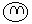\$B8D?ME*\$K\$O(BBugBrowser\$B\$_\$?\$\$\$J46\$8\$,9%\$_\$J\$N\$G!"\$A\$H%+%9%?%^%\$%:\$7\$h\$&\$+\$J!#(B
\$B\$"\$s\$^\$j%?%V\$r3+\$-\$9\$.\$k\$H!"#12hLL\$N2#I}\$KI=<(\$7\$-\$l\$J\$/\$J\$k\$1\$I(B(\$B4@(B;)\$B!#(B
unix.js\$BCf\$N:G>.%U%)%s%H%5%\$%:\$N;XDj\$rJQ99\$7\$F!"%a%K%e!<\$J\$I\$G;H\$o\$l\$k%U%)%s%H%5%\$%:\$r>.\$5\$/\$9\$k5;\$,;H\$(\$J\$/\$J\$C\$F\$\$\$k\$h\$&\$@!#(B
mozilla composer\$B\$N(BSave As Charset\$B\$K\$F!"(BCharset\$B\$,A*Br\$G\$-\$J\$/\$J\$C\$F\$7\$^\$C\$?!#(B
mozilla composer\$B\$N(BSave\$B;~(BHTML reformat(pretty print)\$B;~\$K(Bdl - dt - dd - /dl \$B\$N%\$%s%G%s%H\$,68\$&\$N\$OD>\$C\$F\$\$\$J\$\$!#(B

GAME::\$B??;0T"L5AP#2(B::\$B\$O\$8\$a\$?\$h(B

\$B9XF~\$7\$F\$"\$C\$???;0T"L5AP#2\$r%W%l%\$!#(B
\$B#12sL\\$O!"%W%l!<%d!<%-%c%i%/%?;`K4\$G%2!<%`%*!<%P!<(B(\$B4@(B;)\$B!#(B
\$B#22sL\\$O%W%l!<%d!<%-%c%i%/%?0J30A4LG\$G%2!<%`%*!<%P!<\$H\$\$\$&L5;4\$JGTKL\$r5J\$9\$k\$,!"\$@\$s\$@\$s%3%D\$,F]\$_9~\$a\$F\$-\$?!#(B
\$B\$3\$j\$cLLGr\$\$\$o!*(B\$B0l8@\$G8@\$&\$H(B
\$B0l53Ev@i\$N%?%/%F%#%/%9(B(\$B8x<0%Z!<%8\$h\$j0zMQ(B)
\$B%W%l!<%d!<\$,A`:n\$9\$kIp>-\$OK\Ev\$K0l53Ev@i!#(B
\$B#1\$D\$N%^%C%W\$GE]\$9E(\$N?t\$O(B300\$B\$H\$+!"B?\$\$;~\$K\$O(B700\$B\$r1[\$(\$k!#(B
\$B@oF.;~4V\$O(B15\$BJ,!A(B40\$BJ,\$/\$i\$\$!#(B
\$BC1\$J\$k0u>]\$J\$N\$@\$,!"E(A473\$N(B25%\$BDxEY\$O0l?M\$GE]\$7\$F\$\$\$k\$N\$G\$O\$J\$+\$m\$&\$+(B(\$B4@(B;)\$B!#(B

\$B%?%/%F%#%/%9(B(\$B@o=Q(B)
\$B1&>e\$K%^%C%W\$,I=<(\$5\$l\$F\$*\$j!"@o>l\$G\$N%K%e!<%9\$O%K%e!<%9B.Js\$N\$h\$&\$J7A\$GJs9p\$5\$l\$k!#(B
\$BL#J}\$NK\?X\$,96\$a9~\$^\$l\$?\$i%@%C%7%e\$GLa\$C\$FE(\$r]SLG\$7!"L#J}\$N@hK/\$,Jx\$l\$+\$1\$?\$i%@%C%7%e\$G?J\$s\$GE(\$r]SLG\$9\$k!#(B
\$B%W%l!<%d!-\$J\$N\$G!"%W%l!<%d!<\$,%^%C%W>e\$N\$I\$3\$G@o\$C\$F\$\$\$k\$+\$K\$h\$C\$F@o\$NN.\$l\$,JQ\$o\$C\$F\$/\$k!#(B
\$B\$I\$&\$G\$bNI\$\$=j\$GJ3@o\$7\$F\$\$\$F\$O%@%a!#(B

\$B%-%c%iA*Br(B
\$B;09q;V\$NM-L>%I%3%m\$+\$i\$o\$1\$N\$o\$+\$i\$s(B(?)\$B=w\$N;R\$^\$G(B12\$B?M\$+\$iA*Br2DG=!#(B
(\$B#1<~%/%j%"\$9\$k\$H!"A*Br2DG=%-%c%i\$,A}\$(\$k\$i\$7\$\$!#(B)
\$B\$H\$j\$"\$(\$:=w\$N;R%-%c%i\$rA*Br\$7\$F\$_\$^\$7\$?(B(\$B\$)!#(B
\$B;09q;V%2!<%`\$G\$I!<\$G\$b\$\$\$\$%-%c%i\$rA*Br\$9\$k\$J\$s\$6!"\$+\$J\$j%@%a\$G\$9\$J!D!#(B

\$B@oF.J}<0(B
\$B54IpuBV\$G!{\$r2!\$9\$HBg5;\$,_ZNv\$7\$?\$j\$9\$k!#(B
\$B;(J<\$N967bIQEY\$O\$=\$l\$[\$I9b\$/\$J\$/!"<+J,\$,F0\$-2s\$C\$F\$\$\$l\$P\$^\$:967b\$O-\$N967bIQEY\$O\$=\$l\$J\$j\$K9b\$\$\$N\$G!"??LLL\\$KAj-;0?M\$K0O\$^\$l\$?\$i!"\$H\$j\$"\$(\$:F(\$2\$?J}\$,\$\$\$\$!#(B
\$B5U\$K!"L#J}\$NIp>-\$N6a\$/\$G@oF.\$7\$F\$\$\$k\$H3Z\$A\$s!#(B

\$B@oF.\$NJ70O5\$(B
\$B;(J<\$d\$iIp>-\$d\$i\$,E(L#J}\$"\$o\$;\$F(B5\$B!A(B40\$B?M\$/\$i\$\$\$G!"@o>l\$N\$"\$A\$3\$A\$G%i%0%S!<\$G\$\$\$&=j\$N%b!<%k\$r:n\$C\$F\$*\$j!"\$o\$5\$o\$5F0\$-2s\$C\$?\$jBPV5\$7\$?\$j\$7\$F\$\$\$k!#(B
\$B\$3!<\$f!<\$N9%\$-\$@\$J!A!#(BPSO\$B\$G8@\$&\$HL488#4\$K6a\$\$L)EY\$GE(\$,\$\$\$k\$,!"E(\$HL#J}\$O4qNo\$K%b!<%k\$r:n\$C\$FBPV5\$7\$F\$\$\$k\$"\$?\$j\$,\$A\$g\$C\$H0c\$&!#(B
\$B%W%l!<%d!<\$,A`:n\$9\$k%-%c%i%/%?!<\$O!"%b!<%k\$NCf\$KHt\$S9~\$s\$G@Z\$j\$D\$1\$^\$/\$k46\$8\$K\$J\$k!#(B
\$B<+J,\$NIp4o\$O967bHO0OFb\$J\$iJ#?t\$NE(\$K%R%C%H\$9\$k\$N\$G!"\$&\$^\$/Ht\$S9~\$a\$P\$R\$H?6\$j\$G(B4,5\$B?M\$O?a\$-Ht\$P\$;\$k!#(B
(\$BL#J}\$K\$O967b\$O%R%C%H\$7\$J\$\$\$N\$G!"0B?4\$7\$FE(L#J}\$,F~\$j:.\$8\$C\$?%b!<%k\$NCf\$G%V%s%V%s?6\$j2s\$;\$k!#(B)
\$B%3%s%\\$,7h\$^\$l\$P(B15\$B?M\$O?a\$-Ht\$V!#(B
\$B

RPG\$BMWAG(B - \$BIp7.%]%\$%s%H(B
\$BE(\$rE]\$9!"E(\$NIp>-\$rF\$\$A\$H\$k!"L\I8%?%\$%`\$h\$j\$bAa\$/%^%C%W\$r96N,\$9\$k\$J\$I\$GIp7.%]%\$%s%H\$,\$b\$i\$(\$k!#(B
\$B\$3\$l\$G<+J,\$N%-%c%i%/%?\$N0L\$,\$"\$,\$k!#(B
\$B\$^\$?!"<+J,\$,0z\$-O"\$l\$F\$\$\$k=>B4(B(?)\$B\$b0L\$,\$"\$,\$k\$H?M?t\$,#2?M\$+\$i#4!"#6?M!":G=*E*\$K\$O#8?M\$KA}\$(\$?\$j!"\$I\$s\$I\$s6/\$/\$J\$k\$N\$GJXMx!#(B
(\$B:#\$G\$O%Q%i%a!<%?E*\$K=>B4\$NJ}\$,6/\$/\$J\$C\$F\$7\$^\$C\$?\$h(B.....\$B!#Mj\$`!"E(Ip>-\$r>!RPG\$BMWAG(B - \$B%"%\$%F%`(B
\$BE(\$rE]\$7\$?\$j!"%^%C%W>e\$NH"\$r2u\$9\$H%"%\$%F%`\$,=P8=\$9\$k\$3\$H\$,\$"\$k!#(B
\$BBNNO%Q%i%a!<%?A}6/%"%\$%F%`\$,\$=\$3\$=\$3=P8=\$9\$k\$N\$G!"<+%-%c%i\$O=y!9\$K6/\$/\$J\$C\$F9T\$/!#(B
\$B\$^\$?!"%^%C%W96N,8e\$K\$O3F\$B:\$\$C\$?;v(B

\$B@oF.Cf\$N%b!<%k\$K\$F!"L#J}(B30\$B?ME(0l?M\$N%b!<%k\$K\$J\$C\$F\$\$\$k\$H!"L#J}\$N0~\$K1#\$l\$FE(\$,\$I\$3\$K\$\$\$k\$N\$+8+\$(\$J\$\$(B(\$B4@(B;)\$B!#(B
\$B;~!9@oF.Cf\$N%b!<%k\$,Bg\$-\$/@.D9\$7\$9\$.\$F!"<~0O\$K(B50\$B?M\$[\$I\$N%-%c%i\$,=8\$^\$C\$F\$/\$k\$3\$H\$,\$"\$k!#(B
\$B\$3\$&\$J\$k\$H%b!<%kFb\$J\$s\$@\$1\$I\$A\$g\$C\$H1s\$/\$K5o\$kE(\$,IA2h\$5\$l\$J\$/\$J\$j!"%l!<%@!<\$r8+\$J\$,\$i%l!<%@!<\$BE(L#J}\$N<1JL\$,\$A\$g\$C\$HFq\$7\$\$!#(B
\$BE(\$,\$"\$+\$i\$5\$^\$K%(%\$%j%"%s\$@\$C\$?(BPSO\$B\$HHf3S\$9\$k\$H!"I~Au\$,0c\$&\$@\$1\$N?M4VF1;N\$N@oF.\$@\$7!D!#(B
\$BIp4o%"%\$%F%`\$,F~H\$7\$J\$\$\$H!D!#(B

\$BC:aT(B

\$BM9JXJ*\$GC:aT\$O%\$%d\$s!#(B
\$B\$7\$+\$7!"\$3\$&!"DK\$\$%H%3%m\$rFM\$\$\$F\$-\$^\$9\$J!D!#(B

\$B"#(B \$B1'ChNr(B 2001.10.15 (Mon)
http://www.ceres.dti.ne.jp/~george/jdiaryA11001.html#20011015
Visor::\$BEECS@Z\$l\$?(B

P-in Comp@ct\$B\$rA^\$7\$?\$^\$^\$K\$7\$F=5Kv\$:\$C\$HJ|CV\$7\$F\$\$\$?\$i!"EECS\$,@Z\$l\$FFbMF\$,A4It>C\$(\$F\$\$\$?\$j!#(B\$B\$^\$!!"KhD+(BSync\$B\$7\$F\$\$\$k\$+\$i!">C\$(\$?\$N\$O6bMKF|\$N%G!<%?\$@\$1\$J\$s\$@\$1\$I!#(B

GAME::\$B??;0T"L5AP#2(B::\$B%/%j%"\$7\$?\$h(B

\$BL5AP%b!<%I(B(\$B%9%H!<%j!<%b!<%I\$@\$H;W\$\$\$M\$'(B)\$B\$r\$A\$g\$C\$H?J\$a\$F!"2?EY\$bM7\$Y\$FA}2CJ,\$N%"%\$%F%`\$d%9%F!<%?%9\$OL5AP%b!<%I\$K;}\$A1[\$;\$k%U%j!<%b!<%I\$G=\$9T\$7\$F!"(BEASY\$B!&L5AP%b!<%I!"B9>09a%/%j%"!#(B
\$B%/%j%"\$7\$F\$_\$k\$H0l=V\$@\$C\$?\$J!A!#(B
\$B\$7\$+\$7\$^\$!!"!VB9>09a!W\$J\$s\$F\$f!09a\$GO\$I[\$H@o\$C\$F\$_\$?\$b\$N\$N!"O\$I[\$O\$a\$A\$c\$/\$A\$c6/\$+\$C\$?!D!#(B
\$BB.96\$GJV\$jF\$\$A!u%2!<%`%*!<%P!

\$B%-%c%i46A[\$J\$I(B
\$B9q(B  \$BIp>-(B    \$B0u>]\$J\$I(B
\$B8b(B  \$BB9>09a(B  \$BDL>o967b(B  \$B967b%9%T!<%I\$,Aa\$/!"%3%s%\Cf\$K3d\$j9~\$^\$l\$k\$3\$H\$O>/\$J\$\$!#(B\$B967b\$N\$B967bNO\$O\$"\$s\$^\$j9b\$/\$J\$\$\$h\$&\$@!#(B
\$BAv\$j9~\$_967b(B  \$B\$=\$3\$=\$39-\$\$HO0O\$KL?Cf\$7!"Aj\$B;H\$C\$?8e\$N7d\$O>/\$J\$\$\$h\$&\$@!#(B
\$BL5APMpIq(B  \$B<+J,\$rCf?4\$K(B360\$BEY967b2DG=\$GJXMx!#(B
\$B??L5APMpIq(B  \$B<+J,\$N(BHP\$B\$,>/\$J\$\$;~\$KH/F0!#(B\$B%(%U%'%/%H\$OGI
\$B\$=\$NB>(B  \$BE(Ip>-\$rE]\$7\$?;~\$N@<\$,\$A\$g\$C\$H%P%+\$C\$]\$/\$F!"E(\$NIp>-\$KF1>p\$7\$?\$/\$J\$k(B(\$B\$)!#(B

\$B#2\$A\$c\$s\$M\$k\$N96N,>pJs\$r=8\$a\$?%Z!<%8(B\$B??!&;0T"L5AP#2(B

\$B\$*\$C\$H!#(B
\$B#2%-%c%iJ,L5AP%b!<%I\$r%/%j%"\$7\$J\$\$\$H=P8=\$7\$J\$\$M-L>Ip>-(B(\$B\$H\$\$\$&\$+!"7/

\$BF|5-%U%!%\$%k%j%9%H(B

jdiaryA10816.html (2001.08.16) -
jdiaryA10901.html (2001.09.01) -
jdiaryA10916.html (2001.09.16) -
jdiaryA11016.html (2001.10.16) -

\$B;d\$N:n@.\$7\$?%Z!<%8\$OA4It%j%s%/%U%j!<\$G\$9!#(B
\$B\$3\$N%Z!<%8\$K4V0c\$\$\$dMWK>\$J\$I\$,\$"\$j\$^\$7\$?\$i(B  george@yk.rim.or.jp  \$B\$^\$G8fO"Mm\$/\$@\$5\$\$!#(B

[\$B%[!<%`%Z!<%8(B]
[\$BF|5-(B]
[\$BFI\$s\$G\$\$\$kF|5-(B]
[\$B=)MU862A3J(B]
[FreeBSD]
[\$B8!:w(B]
[\$B9bB.8!:w(B]

home:
<george@yk.rim.or.jp>
or
<george@ceres.dti.ne.jp>

```